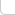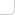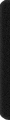# 某島

… : "…アッカリ～ン . .. . " .. .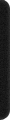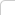January 29, 2013

## Problem D. Wall Bars

### Brief description:

… 。。構造一個層數為 n 的塔。。每一層向四個方向中的一個伸出一個台階。。
。使得可以從地面登上塔頂。。攀爬的最大跨度為 h。。中間不可以換方向。
( n <= 1000, h <= 30 。。。

### Analysis:

。。dp[i][x][y][z][w]：
。。O(n*h4) 狀態。。表示當前第 i 層。。四個方向的最後一塊木板距離當前層的距離分別為 x, y, z, w 時的方案數。。（。。當距離達到 h 時。。高度不再增加。此時的間隙已經過大。。之後不可以參與轉移。。
。。dp[i][x][y][z][w]:
。。O(n*h3) 狀態。。表示當前第 i 層。。上一層是否產生了不可轉移的間隙 w 。。。以及上上一層。。上上上 。的距離分別為分別 z, y, x 時的方案數。。（。。這樣狀態壓縮過後。。x,y,w,z 保存的都是相對位置。。
。。轉移依然是 O(1)。。枚舉四個方向。。。

http://codeforces.com/contest/268/submission/3032299

const int H = 30 + 1;
int dp[H][H][H];
int n, h;

int main(){

#ifndef ONLINE_JUDGE
freopen("in.txt", "r", stdin);
//freopen("out.txt", "w", stdout);
#endif

while (scanf("%d %d", &n, &h) != EOF){
int p = 0, q = 1; int _h = h; ++h; RST(dp[p]); dp[p] = 1;
REP_1(i, n){
swap(p, q); RST(dp[p]);
#define u dp[q][x][y][z][w]
#define t(x) (x==_h?_h:x+1)
#define ww(x) (x==_h)
REP_4(x,y,z,w,h,h,h,2) if (u){
int zz = w ? _h : 1;
INC(dp[p][t(x)][t(y)][t(z)][w], u);
INC(dp[p][t(x)][t(y)][zz][ww(z)], u);
INC(dp[p][t(x)][t(z)][zz][ww(y)], u);
INC(dp[p][t(y)][t(z)][zz][ww(x)], u);
}
}

int res = 0; swap(p, q); REP_4(x,y,z,w,h,h,h,2) if (u){
if (x<_h||y<_h||z<_h||!w) INC(res, u);
}

OT(res);
}
}